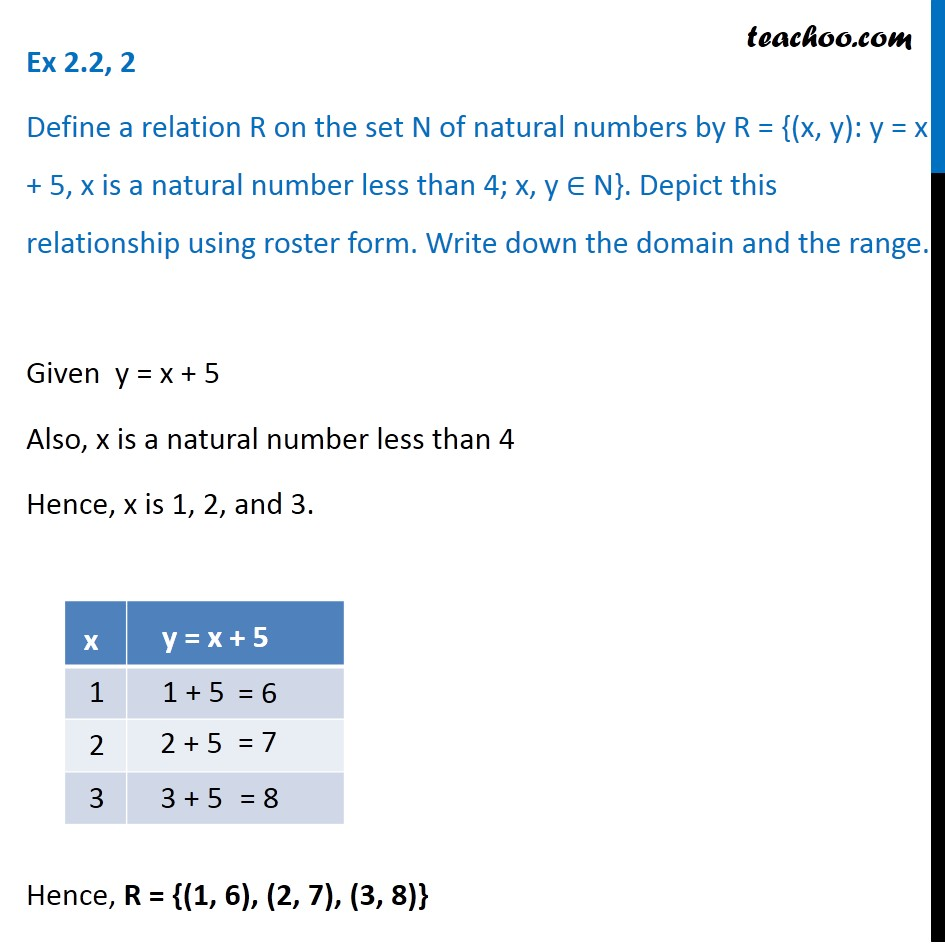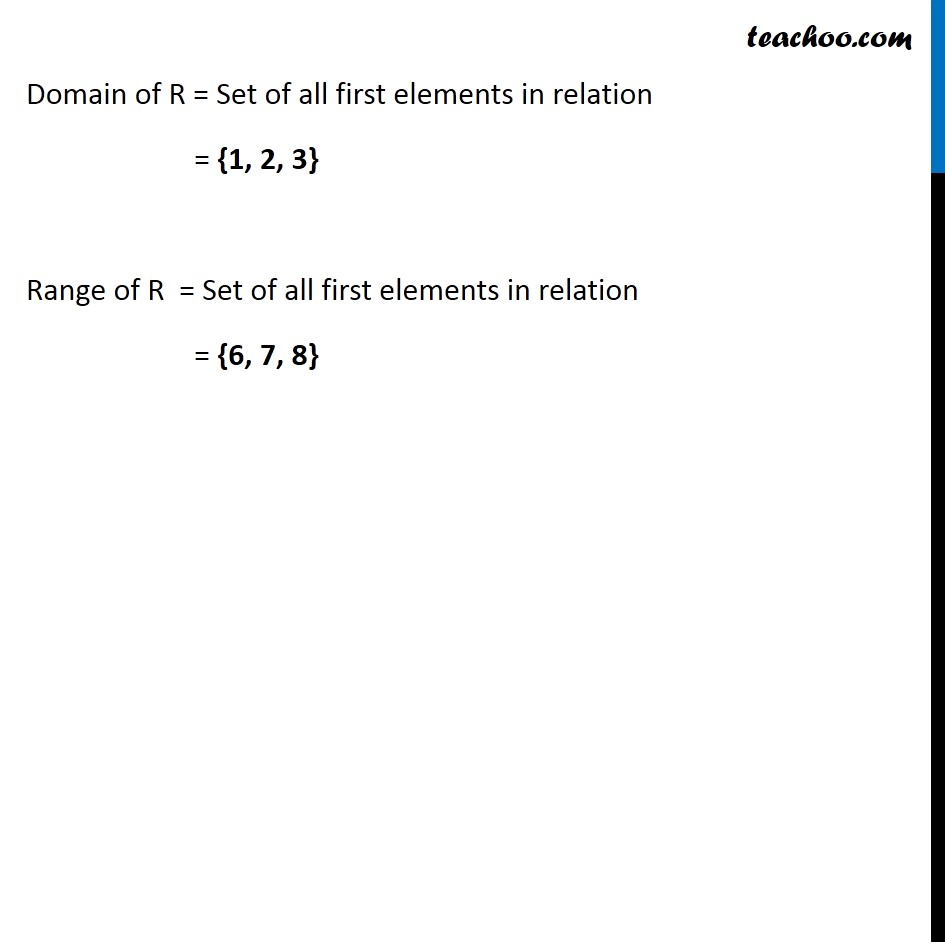Finding Relation - Set-builder form given

Chapter 2 Class 11 Relations and Functions
Concept wiseLearn in your speed, with individual attention - Teachoo Maths 1-on-1 Class

### Transcript

Ex 2.2, 2 Define a relation R on the set N of natural numbers by R = {(x, y): y = x + 5, x is a natural number less than 4; x, y ∈ N}. Depict this relationship using roster form. Write down the domain and the range. Given y = x + 5 Also, x is a natural number less than 4 Hence, x is 1, 2, and 3. Hence, R = {(1, 6), (2, 7), (3, 8)} Domain of R = Set of all first elements in relation = {1, 2, 3} Range of R = Set of all first elements in relation = {6, 7, 8}